# Pairwise Error Probability Analysis of Dual Hop Relaying Network over Time Selective Nakagami-m Fading Channel with Imperfect CSI and Node Mobility

Pairwise Error Probability Analysis of Dual Hop Relaying Network over Time Selective Nakagami-m Fading Channel with Imperfect CSI and Node Mobility

National Institute of Technology Patna, Patna - 800005, India

Corresponding Author Email:
ravishankar@mits.ac.in
Page:
281-295
|
DOI:
https://doi.org/10.18280/ts.360312
4 March 2019
|
Accepted:
28 May 2019
|
Published:
1 September 2019
| Citation

OPEN ACCESS

Abstract:

We investigate the end-to-end performance of the selective decode and forward (S-DF) relaying network over time selective independent but not necessarily identically distributed (i.n.i.d.) Nakagami-m fading channel considering practical communication constraints such as mobile nodes and imperfect channel state information (CSI). The per block average pairwise error probability (PEP) is investigated for both optimal and equal power allocation scenarios. Simulation results have been presented for various values of fading severity parameter and channel variance. The end-to-end performance is examined for various node mobility scenarios and for various antenna configurations at the source, relay and destination nodes. Simulation results confirm the accuracy of the analytical results.

Keywords:

selective decode-and-forward, multiple- input multiple-output, channel state information, diversity order, signal to noise ratio

1. Introduction

Cooperative wireless communication enables efficient use of communication resources, so that user devices can collaborate with each other in wireless transmission in wireless networks. This is a promising technique for the 5th Generation (5G) wireless system . With the advent of the Internet of Things (IoT)  as a promising technique that strives to revolutionize the global world through uninterrupted connectivity of smart devices, vision of Smart Cities is close to a reality. 5G technology will be a potential key technology for future IoT systems. The upcoming 5G technology assures low latency, high reliability, high data rates, uninterrupted connectivity, high throughput and better performance. However, the performance of any system gets worse when such a system fades deeper, which is usually on a single link scenario.

Literature describes the amplify-and-forward (AF)  and decode-and-forward (DF)  cooperative communication protocols as the most popular choices for cooperative relay-based communication due to their robust performance and implementation simplicity. In , the authors investigated the performance of wireless powered relay with AF cooperative communication protocol over frequency flat Nakagami-m fading channels considering the non-linearity of the energy harvester. The closed-form expressions for outage channel capacity is obtained using the complementary cumulative distribution function (CCDF) of the end-to-end signal to noise ratio (SNR). In , the authors investigated the AF relaying protocol over correlated Rayleigh fading channel conditions. In this work outage probability (OP) of best relay selection (BRS) based AF wireless system is investigated considering correlated source to destination (SD), source to relay (SR) and relay to destination (RD) fading links.

In , the authors derived the maximum likelihood decoder for the decode and forward (DF) cooperative communication network using complex valued constellations including M-ary pulse amplitude modulation (M-PAM), M-ary quadrature amplitude modulation (M-QAM) and M-ary phase shift keying (M-PSK). The relay and source nodes employ orthogonal-space time block code (OSTBC) transmissions. A low-complexity piecewise linear decoder is derived for arbitrary complex-valued constellations, which performs like maximum likelihood decoder for all SNR values. However, AF relaying suffers from noise amplification and fixed DF fears performance degradation in case of improper decoding at relay as described in [3-7]. To overcome this problem S-DF relaying protocol is used in which if the SNR of a signal received at the relay exceeds a certain threshold then the relay decodes the received signal and forwards the decoded information to the destination.

In , the authors investigated the symbol error rate (SER) performance of the S-DF relaying network based multiple relayed relaying network for M-QAM signalling. The Q(.) function is used to obtain the closed form (CF) expression of SER and asymptotic-SER over frequency flat Rayleigh fading channel. However, if the channel between the source and the relay suffer a severe fading and the SNR falls below the threshold; the relay remains idle. In the past, some significant works have been done by the researchers on S-DF based cooperative communication systems [9-11]. Further, it is observed that the performance of S-DF protocol-based system can be improved with the use of multiple parallel relay nodes which helps in achieving diversity gain.

In , the authors investigated a secure communication system under multiple attack types such as jamming, spoofing, and eavesdropping from unmanned aerial vehicle (UAV). The real time communication scenarios are considered such as imperfect CSI and limited number of pilot symbols. To deal with the joint impact of imperfect channel estimation and smart attack, a Nash equilibrium (NE) based algorithm is proposed to suppress the smart attack from the UAV. In the work , the authors considered DF based mixed MIMO radio frequency/free space optics (RF/FSO) relaying network for underlay cognitive radio conditions. Consequently, a PEP performance analysis is demonstrated, and CF expressions are derived for the per-frame average OP, considering both orthogonal-STBC transmission and transmit antenna selection (TAS) at the secondary user (SU). The analysis assumes that the RF links between the SU-primary user (PU) and SU-relay are Nakagami-m fading distributed, while the FSO link between the RD eNodeB is assumed to be affected by optical channel impairments, such as atmospheric turbulence, path loss and pointing errors, thus including the important characteristics of both the fading links. The impact of PU node mobility on the PEP and OP performance of the cognitive radio network is investigated in this work and improved OP performance is achieved for the MIMO-RF/FSO DF cognitive relaying network within contrast to single antenna AF systems given in the existing works.

We conclude the following novel contributions from this paper:

The CF per block average PEP, asymptotic PEP and asymptotic error floor limit expressions are derived for MIMO STBC S-DF system over time selective Nakagami-m fading channel, with imperfect CSI, and with node mobility scenario.

Further, a framework is developed for deriving the diversity order (DO) and results are sho ${}_{a}{{F}_{b}}\left( .;.;.;. \right)$ wn for various values of shape parameter.

Notation: The following notations are used for the mathematical expressions presented in this paper. $\left| \Phi \right|,\,\,trace\left\{ \Phi \right\}$ and ${{\Phi }^{H}}$ denote the modulus, trace and conjugate transpose of the matrix $\Phi ,$  respectively. The Euclidean norm or Frobenius norm of matrix $\Phi$  is denoted by $||\Phi |{{|}_{F}}$  , denotes the Gauss hypergeometric function . Expectation operator is denoted by $\text{E}\left\{ . \right\}$ and $\text{Q}\left( u \right)$ denotes the probability that a standard random variable (RV) Y takes a value larger than u, i.e., ${{\operatorname{P}}_{r}}[\mathbf{Y}\ge u]$ . ${{\text{J}}_{0}}(.)$ denotes the Bessel function of the first kind and zeroth-order  and ${{\Phi }^{T}}$ denotes the transpose of the matrix $\Phi .$

The rest of the paper is organized as follows; system model of S-DF cooperation protocol is given in section 2. The DH dual phase single relay S-DF cooperation protocol is investigated in section 3. In this section, we derive the per block average PEP expression over time selective Nakagami-m fading channel with imperfect CSI and node mobility. Also, in this section, Karush-Kuhn-Tucker (KKT)  based convex optimization (CO)  framework is developed for finding the DO and optimal source-relay power allocation factors. Simulation results are shown in section 4. Finally, the paper is concluded in section 5.

2. System Model of Mimo Stbc S-DF Cooperative Communication System

We consider a S-DF cooperative communication system in which relay node is ready to collaborate with the source node in forwarding its signal to the receiving node over orthogonal channels, which could be attained either by code division multiple access (CDMA), frequency division multiple access or time division multiple access (TDMA). Let ND, NR and NS are the number of antennas employed at the destination, relay and source nodes, respectively. In order to keep the data rate of the SR fading link same as that of the RD fading link, we herein propose to use the same Alamouti orthogonal space time block code (OSTBC) at the source and relay, respectively. This also means that, ${{N}_{S}}={{N}_{R}}=N$ [9-12]. Let ${{\tilde{\ell }}^{rd}}_{l,n}(\tau ),$ and $\tilde{\ell }_{\tilde{n},n}^{sr}(\tau )$ denote the instantaneous channel gains for relay transmit antenna to destination receive antenna and Sth  source transmit antenna $\left\{ s\in \left( 1,2,3,.....,{{N}_{S}} \right) \right\}$ to relay received antenna, respectively. Also, the instantaneous channel gain for the fading links from the Sth  source transmit antenna to destination receive antenna is represented by ${{\tilde{\ell }}^{sd}}_{\tilde{l},n}(\tau ).$  The data transmission is divided into two phases in the first phase of the signal transmission source node transmit the Alamouti STBC codeword ${{\text{X}}_{S}}[\tau ]\in \mathbb{C}{{}^{N\times {{T}_{S}}}}$ to relay and destination nodes, respectively. Where ${{\text{T}}_{\text{S}}}$ and $\tau$ represents the number of time slots and ${{\tau }^{th}}$ MIMO STBC code-word in a block of Mb  code-word matrices, respectively [9-11]. The received code-word’s at the destination node, ${{\mathbf{\Psi }}_{SD}}[\tau ]\in {{\mathbb{C}}^{{{N}_{D}}\times {{\text{T}}_{\text{S}}}}}$ and at the relay, ${{\mathbf{\Psi }}_{SR}}[\tau ]\in {{\mathbb{C}}^{N\times {{\text{T}}_{\text{S}}}}},$  corresponding to the MIMO STBC code-word transmission from the source can be written as , , ,

${{\mathbf{\Psi }}_{SD}}[\tau ]=\sqrt{{{P}_{S}}/N{{R}_{C}}}{{\mathbb{N}}_{SD}}[\tau ]{{X}_{S}}[\tau ]+{{Z}_{SD}}[\tau ],$   (1)

${{\mathbf{\Psi }}_{SR}}[\tau ]=\sqrt{{{P}_{S}}/N{{R}_{C}}}{{\mathbb{N}}_{SR}}[\tau ]{{\text{X}}_{S}}[\tau ]+{{Z}_{SR}}[\tau ].$ (2)

where, ${{P}_{S}}$ and ${{R}_{C}}$ denote the available power at the source node and coding rate, respectively. The SD ${{\mathbb{N}}_{SD}}[\tau ]\in \mathbb{C}{{\,}^{{{N}_{D}}\times N}}$ and SR ${{\mathbb{N}}_{SR}}[\tau ]\in {{\mathbb{C}}^{N\times N}}$ channel matrices are comprised of  terms which are statistically independent but not necessarily identically distributed with each other, modeled as uniform phase and Nakagami-m faded envelope with shape parameter, ${{m}_{i}}\ge 0.50;\,\,i\in \left\{ SD,SR \right\}$ and controlling spreads $\delta _{SD}^{2}$ and $\delta _{SR}^{2},$ respectively. The physical phenomenon behind Nakagami-m model is clustering of multipath waves. Clustering of multipath waves yields to the Nakagami-m fading envelope. More precisely, 2m squared zero average value equal-variance Gaussians lead to a Gamma variate with shape parameter m, whose square root is equal to the Nakagami-m fading envelope . In the relaying phase, relay node re-transmits only successfully decoded signal to the destination node, otherwise, it will remain in the idle state. The received symbol blocks at the destination node ${{\mathbf{\Psi }}_{RD}}[\tau ]\in {{\mathbb{C}}^{{{N}_{D}}\times {{\text{T}}_{\text{S}}}}},$ corresponding to transmission from relay node can be written as,

${{\mathbf{\Psi }}_{RD}}[\tau ]=\sqrt{{{P}_{R}}/N{{R}_{C}}}{{\mathbb{N}}_{RD}}[\tau ]{{\text{X}}_{S}}[\tau ]+{{Z}_{RD}}[\tau ].$   (3)

where, ${{P}_{R}}$ denotes the power transmitted from the relay. The RD channel matrix ${{\mathbb{N}}_{RD}}[\tau ]\in {{\mathbb{C}}^{{{N}_{D}}\times N}}$ is comprised of terms, which are statistically independent but not necessarily identically distributed with each other, modeled as uniform phase and Nakagami-m faded envelope with a fading severity or shape parameter, ${{m}_{RD}}\ge 0.50$ and controlling spread $\delta _{RD}^{2}.$ The terms of the channel noise matrices ${{Z}_{RD}}[\tau ],$ ${{Z}_{SR}}[\tau ]$ and ${{Z}_{SD}}[\tau ]$ are statistically independent with each other, modeled as zero mean circular shift complex gaussian (ZMCSCG) RVs with variance $N_0/2$ (per complex dimension), respectively. This work considers mobile nodes, because of this, fading channel links between destination, relay and source nodes become time-selective in nature and can be modeled using AR1 process. Further, it has been considered that the fading is approximately constant over the time interval of a single MIMO STBC code-word while varying in a time-selective manner from one MIMO STBC code-word to another MIMO STBC code-word , . The variation of the SD, SR and RD channel matrix within a block is modeled as , ,

${{\mathbb{N}}_{SD}}[\tau ]={{\rho }_{SD}}{{\mathbb{N}}_{SD}}[\tau -1]+\sqrt{1-\rho _{SD}^{2}}{{\mathbb{Q}}_{SD}}[\tau ],$   (4)

${{\mathbb{N}}_{SR}}[\tau ]={{\rho }_{SR}}{{\mathbb{N}}_{SR}}[\tau -1]+\sqrt{1-{{({{\rho }_{SR}})}^{2}}}{{\mathbb{Q}}_{SR}}[\tau ],$  (5)

${{\mathbb{N}}_{RD}}[\tau ]={{\rho }_{RD}}{{\mathbb{N}}_{RD}}[\tau -1]+\sqrt{1-{{({{\rho }_{RD}})}^{2}}}{{\mathbb{Q}}_{RD}}[\tau ].$  (6)

The SD, SR and RD fading link’s correlation coefficients are denoted by ${{\rho }_{SD}},\,\,{{\rho }_{SR}}$ and ${{\rho }_{RD}},$ respectively. We can evaluate the correlation coefficients by using classical Jake's model , given as, $\rho ={{\text{J}}_{0}}(2\pi {{f}_{C}}{{v}_{p}}/{{R}_{S}}\text{c}).$ Where ${{f}_{C}},{{v}_{p}},{{R}_{S}}$ and c denote the carrier frequency, relative velocity between two communicating nodes, symbol transmission rate and velocity of light, respectively. The SD, SR and RD random processes are denoted as ${{\mathbb{Q}}_{SD}}[\tau ],\,\,{{\mathbb{Q}}_{SR}}[\tau ]$ and ${{\mathbb{Q}}_{RD}}[\tau ],$ respectively, distributed as ZMCSCG, with variances $\sigma _{eSD}^{2},\sigma _{eSR}^{2}$ and $\sigma _{eRD}^{2},$ respectively . This work considers that the receiving node is employed by the low complexity maximal ratio combiner (MRC) receiver ,  but due to time-selective nature of the fading channel links it is tough to obtain the knowledge of instantaneous CSI corresponding to the transmission of MIMO STBC code-word. Hence, in this paper, like the works -, imperfect CSI is considered at the relay and destination nodes. The estimated channel matrices for RD, SR and SD links can be modeled as ,

$\underbrace{{{{\hat{\mathbb{N}}}}_{RD}}}_{\text{ESTIMATED}\,\,\text{CHANNEL}\,\,\text{MATRIX}}=\underbrace{{{\mathbb{N}}_{RD}}}_{\text{CHANNEL}\,\,\text{MATRIX}}+\underbrace{{{\mathbb{N}}_{\in RD}}}_{\text{CHANNEL}\,\,\text{ERROR}\,\,\text{MATRIX}},$

$\underbrace{{{{\hat{\mathbb{N}}}}_{SR}}}_{\text{ESTIMATED}\,\,\text{CHANNEL}\,\,\text{MATRIX}}=\underbrace{{{\mathbb{N}}_{SR}}}_{\text{CHANNEL}\,\,\text{MATRIX}}+\underbrace{{{\mathbb{N}}_{\in SR}}}_{\text{CHANNEL}\,\,\text{ERROR}\,\,\text{MATRIX}},$

\begin{align} & \text{and,}\underbrace{{{{\hat{\mathbb{N}}}}_{SD}}}_{\text{ESTIMATED}\,\,\text{CHANNEL}\,\,\text{MATRIX}}=\underbrace{{{\mathbb{N}}_{SD}}}_{\text{CHANNEL}\,\,\text{MATRIX}} \\ & +\underbrace{{{\mathbb{N}}_{\in SD}}}_{\text{CHANNEL}\,\,\text{ERROR}\,\,\text{MATRIX}}, \\ \end{align}  (7)

respectively, estimated at the beginning of each block and in this way used to detect each MIMO STBC code-word ${{X}_{S}}[\tau ],1\le s\le {{M}_{b}}$ in the consequent block . The channel error matric ${{\mathbb{N}}_{\in SR}},\,\,{{\mathbb{N}}_{\in SD}}$ and ${{\mathbb{N}}_{\in RD}}$ comprise of entries, which are ZMCSCG with variance $\sigma _{\in SR}^{2},\sigma _{\in SD}^{2}$ and $\sigma _{\in RD}^{2},$ respectively.  By using (4), ${{\mathbb{N}}_{SD}}[\tau ]$ can be modeled as , ,

\begin{align} & {{\mathbb{N}}_{SD}}[\tau ]=\rho _{SD}^{\tau -1}{{{\hat{\mathbb{N}}}}_{SD}}-\rho _{SD}^{\tau -1}{{\mathbb{N}}_{\in SD}}+ \\ & \sqrt{1-\rho _{SD}^{2}}\sum\limits_{i=1}^{\tau -1}{\rho _{SD}^{\tau -i-1}{{\mathbb{Q}}_{SD}}[i]}. \\ \end{align} (8)

Substituting the expression of ${{\mathbb{N}}_{SD}}[\tau ]$ given above in (1), ${{\mathbf{\Psi }}_{SD}}[\tau ]$ can be written as , -,

${{\mathbf{\Psi }}_{SD}}[\tau ]=\underbrace{\sqrt{{{P}_{S}}/N{{R}_{C}}}\rho _{SD}^{\tau -1}\hat{\mathbb{N}}{{X}_{S}}[\tau ]}_{\text{DESIRED}\,\,\,\text{SIGNAL}}+\underbrace{Z_{SD}^{Eff}}_{\text{EFFECTIVE NOISE MATRIX}\,\,}.$  (9)

where, effective noise matrix for SD fading link $Z_{SD}^{Eff}$ is given below ,

\begin{align} & Z_{SD}^{Eff}=\overbrace{{{Z}_{SD}}[\tau ]}^{\text{WHITE }\,\text{NOISE}}+\overbrace{\sqrt{{{P}_{S}}(1-\rho _{SD}^{2})/N{{R}_{C}}}\sum\limits_{i=1}^{\tau -1}{\rho _{SD}^{\tau -i-1}}{{\mathbb{Q}}_{SD}}(i){{\text{X}}_{S}}[\tau ]}^{\text{NOISE}\,\,\text{TERM}\,\,\text{ARISES}\,\,\text{DUE}\,\,\text{TO}\,\,\text{NODE}\,\,\text{MOBILITY}} \\ & \,\,\,\,\,\,\,\,\,\,\,\,\,-\overbrace{\sqrt{{{P}_{S}}/N{{R}_{C}}}\rho _{SD}^{\tau -1}\,{{\mathbb{N}}_{\in SD}}{{\text{X}}_{S}}[\tau ]}^{\text{NOISE}\,\,\text{TERM}\,\,\text{ARISES}\,\,\text{DUE}\,\,\text{TO}\,\,\text{IMPERFECT}\,\,\text{CSI}}. \\ \end{align}

The effective noise variance ${{\eta }_{SD}}$ of effective noise matrix $Z_{SD}^{Eff}$ is given as , ,

\begin{align} & {{\eta }_{SD}}=\overbrace{{{N}_{0}}}^{\begin{smallmatrix} \text{WHITE }\,\text{NOISE } \\ \text{ VARIANCE} \end{smallmatrix}}+\overbrace{({{P}_{S}}/N{{R}_{C}})\rho _{SD}^{2(\tau -1)}{{N}_{a}}\sigma _{_{{{\in }_{SD}}}}^{2}}^{\begin{smallmatrix} \text{VARIANCE OF THE NOISE}\,\,\text{TERM}\, \\ \text{ARISES}\,\,\text{DUE}\,\,\text{TO}\,\,\text{IMPERFECT}\,\,\text{CSI}\end{smallmatrix}} \\ & \,\,\,\,\,\,\,\,\,\,\,\,\,\,\,\,\,-\overbrace{({{P}_{S}}/N{{R}_{C}})\left( 1-\rho _{SD}^{2(\tau -1)} \right){{N}_{a}}\sigma _{_{{{e}_{SD}}}}^{2}}^{\begin{smallmatrix} \text{VARIANCE OF THE NOISE}\,\,\text{TERM}\,\, \\ \text{ARISES}\,\,\text{DUE}\,\,\text{TO}\,\,\text{NODE}\,\,\text{MOBILITY}\end{smallmatrix}}. \\\end{align}  (10)

where, ${{N}_{a}}$ denotes the number of non-zero M-ary PSK symbols transmitted per MIMO STBC codeword. Following the similar approach, the received symbol blocks at the relay and destination nodes, respectively, can be expressed as,

\begin{align} & {{\mathbf{\Psi }}_{SR}}[\tau ]=\underbrace{\sqrt{{{P}_{S}}/N{{R}_{C}}}{{({{\rho }_{SR}})}^{\tau -1}}{{{\hat{\mathbb{N}}}}_{SR}}{{X}_{S}}[\tau ]}_{\text{DESIRED}\,\,\,\text{SIGNAL}} \\ & +\underbrace{Z_{SR}^{Eff}}_{\text{EFFECTIVE NOISE MATRIX}\,\,}. \\ \end{align}  (11)

\begin{align} & {{\mathbf{\Psi }}_{RD}}[\tau ]=\underbrace{\sqrt{{{{\tilde{P}}}_{R}}/N{{R}_{C}}}{{({{\rho }_{RD}})}^{\tau -1}}{{{\hat{\mathbb{N}}}}_{RD}}{{X}_{S}}[\tau ]}_{\text{DESIRED}\,\,\,\text{SIGNAL}} \\ & +\underbrace{Z_{RD}^{Eff}}_{\text{EFFECTIVE NOISE MATRIX}\,\,}. \\ \end{align}  (12)

where,

\left\{ \begin{align} & {{{\tilde{P}}}_{R}}={{P}_{R}};\,\,\,\,\,\,\,\,\text{if}\,\text{relay}\,\text{decodes}\,\,\text{the}\,\,\text{symbol}\,\,\text{correcly} \\ & {{{\tilde{P}}}_{R}}=0;\,\,\,\,\,\,\,\,\,\text{if}\,\,\text{relay}\,\text{decodes}\,\,\text{the}\,\,\text{symbol}\,\,\,\text{incorrecly}. \\ \end{align} \right.  (13)

where, effective noise matrix for SR and RD fading link, $Z_{SR}^{Eff}$ and $Z_{RD}^{Eff}$, respectively, are given below,

\begin{align} & Z_{SR}^{Eff}=\overbrace{{{Z}_{SR}}[\tau ]}^{\text{WHITE}\,\,\text{NOISE}}+\overbrace{\sqrt{{{P}_{S}}(1-{{({{\rho }_{SR}})}^{2}})/N{{R}_{C}}}\sum\limits_{i=1}^{\tau -1}{{{({{\rho }_{SR}})}^{\tau -i-1}}}{{\mathbb{Q}}_{SR}}[i]{{\text{X}}_{S}}[\tau ]}^{\text{NOISE}\,\,\text{TERM}\,\,\text{ARISES}\,\,\text{DUE}\,\,\text{TO }\,\text{NODE}\,\,\text{MOBILITY}} \\ & \,\,\,\,\,\,\,\,\,\,\,\,\,\,\,\,\,-\overbrace{\sqrt{{{P}_{S}}/N{{R}_{C}}}{{({{\rho }_{SR}})}^{\tau -i-1}}\,{{\mathbb{N}}_{\in SR}}{{\text{X}}_{S}}[\tau ]}^{\text{NOISE}\,\,\text{TERM}\,\,\text{ARISES}\,\,\text{DUE}\,\,\text{TO}\,\,\text{IMPERFECT}\,\,\text{CSI}}. \\ \end{align}

\begin{align} & Z_{RD}^{Eff}=\overbrace{{{Z}_{RD}}[\tau ]}^{\text{WHITE}\,\,\text{NOISE}}+\overbrace{\sqrt{{{{\tilde{P}}}_{R}}(1-{{({{\rho }_{RD}})}^{2}})/N{{R}_{C}}}\sum\limits_{i=1}^{\tau -1}{{{({{\rho }_{RD}})}^{\tau -i-1}}}{{\mathbb{Q}}_{RD}}[i]{{\text{X}}_{S}}[\tau ]}^{\text{NOISE}\,\,\text{TERM}\,\,\text{ARISES}\,\,\text{DUE}\,\,\text{TO }\,\text{NODE}\,\,\text{MOBILITY}} \\ & \,\,\,\,\,\,\,\,\,\,\,\,\,\,\,\,\,-\overbrace{\sqrt{{{{\tilde{P}}}_{R}}/N{{R}_{C}}}{{({{\rho }_{RD}})}^{\tau -i-1}}\,{{\mathbb{N}}_{\in RD}}{{\text{X}}_{S}}[\tau ]}^{\text{NOISE}\,\,\text{TERM}\,\,\text{ARISES}\,\,\text{DUE}\,\,\text{TO}\,\,\text{IMPERFECT}\,\,\text{CSI}}. \\ \end{align}

The effective noise variances ${{\eta }_{SR}}$ and ${{\eta }_{RD}}$ of $Z_{SR}^{Eff}$ & $Z_{RD}^{Eff}$, respectively, are given below , [16-17],

\begin{align} & {{\eta }_{SR}}=\overbrace{{{N}_{0}}}^{\text{WHITE }\,\text{NOISE VARIANCE}}+\overbrace{({{P}_{S}}/N{{R}_{C}}){{({{\rho }_{SR}})}^{2(\tau -1)}}{{N}_{a}}{{({{\sigma }_{\in SR}})}^{2}}}^{\begin{smallmatrix} \text{VARIANCE OF THE NOISE}\,\,\text{TERM } \\ \text{ARISES}\,\,\text{DUE}\,\,\text{TO}\,\,\text{IMPERFECT}\,\,\text{CSI}\, \end{smallmatrix}}-\, \\ & \overbrace{({{P}_{S}}/N{{R}_{C}})\left( 1-{{({{\rho }_{SR}})}^{2(\tau -1)}} \right){{N}_{a}}{{({{\sigma }_{eSR}})}^{2}}}^{\begin{smallmatrix} \text{ VARIANCE OF THE NOISE}\,\,\text{TERM } \\ \text{ ARISES}\,\,\text{DUE}\,\,\text{TO}\,\,\text{NODE}\,\text{MOBILITY} \end{smallmatrix}}\,, \\ \end{align} (14)

\begin{align} & {{\eta }_{RD}}=\overbrace{{{N}_{0}}}^{\begin{smallmatrix} \text{WHITE }\,\text{NOISE } \\ \text{ VARIANCE}\end{smallmatrix}}+\overbrace{({{P}_{R}}/N{{R}_{C}}){{({{\rho }_{RD}})}^{2(\tau -1)}}{{N}_{a}}\sigma _{\in RD}^{2}}^{\,\text{VARIANCE OF THE NOISE}\,\,\text{TERM}\,\text{ARISES}\,\,\text{DUE}\,\,\text{TO}\,\,\text{IMPERFECT}\,\,\text{CSI}}- \\ & \,\overbrace{({{P}_{R}}/N{{R}_{C}})\left( 1-{{({{\rho }_{RD}})}^{2(\tau -1)}} \right){{N}_{a}}{{(\sigma _{eRD}^{2})}^{2}}}^{\text{ VARIANCE OF THE NOISE}\,\,\text{TERM}\,\text{ARISES}\,\,\text{DUE}\,\,\text{TO}\,\,\text{NODE}\,\,\text{MOBILITY}}. \\ \end{align}  (15)

3. Single Relay Dual Phase Dual Hop MIMO STBC S-DF Relaying Network

3.1 PEP analysis

In this section, per-block average PEP expression is derived for the dual phase DH single relay MIMO-STBC S-DF relaying network. The schematic representation of single relay S-DF protocol is shown in Figure 1. Let MIMO STBC code-word set is denoted by $\text{C}=\left\{ {{\text{X}}_{S}}[\tau ] \right\};1\le s\le \left| \text{C} \right|,$ where $\left| \text{C} \right|$ denotes the cardinality of the set $\text{C}$. The average PEP for the error event ${{\text{X}}_{S}}[\tau ]\to {{\text{X}}_{l}}[\tau ]$, when at the relay node, MIMO STBC code-word ${{X}_{S}}[\tau ]\in {{\mathbb{C}}^{N\times {{\text{T}}_{\text{S}}}}}$ confused for the MIMO STBC code-word ${{X}_{l}}[\tau ]\in {{\mathbb{C}}^{N\times {{\text{T}}_{\text{S}}}}},$ where $l\ne s,$ can be expressed using (2) as ,

## 1.png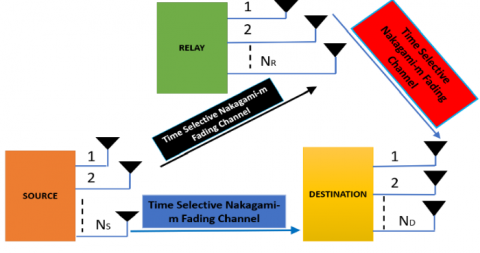Figure 1. Schematic representation of DH dual phase single relay S-DF cooperative communication protocol

\begin{align} & P_{E}^{S\to R}\left( \left. {{X}_{S}}[\tau ]\to {{X}_{l}}[\tau ] \right|{{{\hat{\mathbb{N}}}}_{SR}} \right)= \\ & \,\,\,\,\,\,\,a\text{Q}\left( \sqrt{\frac{b{{P}_{S}}{{({{\rho }_{SR}})}^{2(\tau -1)}}\left\| {{{\hat{\mathbb{N}}}}_{SR}}({{X}_{S}}[\tau ]-{{X}_{l}}[\tau ]) \right\|_{F}^{2}}{2N{{R}_{C}}{{\eta }_{SR}}}} \right) \\ & \,\,\,\,\,\,-\,\,\,c{{\text{Q}}^{2}}\left( \sqrt{\frac{b{{P}_{S}}{{({{\rho }_{SR}})}^{2(\tau -1)}}\left\| {{{\hat{\mathbb{N}}}}_{SR}}({{X}_{S}}[\tau ]-{{X}_{l}}[\tau ]) \right\|_{F}^{2}}{2N{{R}_{C}}{{\eta }_{SR}}}} \right). \\ \end{align}   (16)

where, $\text{Q}(\Phi )$ {\displaystyle Q(x)}is defined as ,

$\text{Q}(\Phi )=\frac{1}{\pi }\int\limits_{0}^{\pi /2}{\exp \left( \frac{-\,{{\Phi }^{2}}}{2{{\sin }^{2}}\psi } \right)d\psi };\,\,\text{for BPSK transmission}.$  (17)

where, a, b, and c are modulation dependent parameters listed in Table 1 . Like works [9, 12, 16, 17] by using the singular value decomposition (SVD) of the MIMO STBC codeword difference matrix ${{X}_{S}}[\tau ]-{{X}_{l}}[\tau ],$ conditional PEP for the SR fading link can be written as,

Table 1. Modulation dependent parameters for various modulation schemes 

 Modulation schemes a b c M-PSK 2 $\text{2si}{{\text{n}}^{\text{2}}}\text{(}\frac{\pi }{\text{M}}\text{)}$ 0 BPSK 1 2 0 BFSK 1 1 0 M-PAM $\text{2}\frac{\text{(M-1)}}{\text{M}}$ $\frac{\text{6}}{{{\text{M}}^{\text{2}}}\text{-1}}$ 0 MSK or QPSK 2 2 1 Coherent DPSK 2 2 2 M-QAM $4\frac{\text{(}\sqrt{\text{M}}\text{-1)}}{\sqrt{\text{M}}}$ $\frac{3}{\text{M}-1}$ $4{{\left( \frac{\sqrt{\text{M}}\text{-1}}{\sqrt{\text{M}}} \right)}^{2}}$

\begin{align} & P_{E}^{S\to R}\left( \left. {{\text{X}}_{S}}[\tau ]\to {{\text{X}}_{l}}[\tau ] \right|{{{\tilde{\mathbb{N}}}}_{SR}} \right)= \\ & a\text{Q}\left( \sqrt{\frac{b{{P}_{S}}{{({{\rho }_{SR}})}^{2(\tau -1)}}\sum\limits_{n=1}^{N}{\lambda _{\ln }^{2}\sum\limits_{\tilde{n}=1}^{N}{{{\left| \tilde{\ell }_{\tilde{n},n}^{sr}(1) \right|}^{2}}}}}{2N{{R}_{C}}{{\eta }_{SR}}}} \right) \\ & \,\,-c{{\text{Q}}^{2}}\left( \sqrt{\frac{b{{P}_{S}}{{({{\rho }_{SR}})}^{2(\tau -1)}}\sum\limits_{n=1}^{N}{\lambda _{\ln }^{2}\sum\limits_{\tilde{n}=1}^{N}{{{\left| \tilde{\ell }_{\tilde{n},n}^{sr}(1) \right|}^{2}}}}}{2N{{R}_{C}}{{\eta }_{SR}}}} \right). \\ \end{align} (18)

where, ${{\lambda }_{l1}},{{\lambda }_{l2}},....,{{\lambda }_{lN}}$ represents the singular values (SVs) obtained after performing SVD of the MIMO STBCcodeword difference matrix ${{X}_{S}}[\tau ]-{{X}_{l}}[\tau ],\tilde{\ell }_{\tilde{n},n}^{sr}(1)$ are the complex Nakagami-m channel coefficients of the resulting SR channel matrix ${{\tilde{\mathbb{N}}}_{SR}}={{\hat{\mathbb{N}}}_{SR}}{{U}_{l}}$ for $1\le \tilde{n},n\le N,$ and ${{U}_{l}}\in {{\mathbb{C}}^{N\times N}}$ is a unitary matrix, i.e., $U_{l}^{H}{{U}_{l}}={{U}_{l}}U_{l}^{H}={{I}_{N\times N}}.$ For Alamouti MIMO STBC or any other orthogonal space-time code, ${{\lambda }_{l1}}={{\lambda }_{l2}}=....={{\lambda }_{lN}}=\lambda .$ Using the identity given in (17), the instantaneous PEP for SR fading link can be written as,

\begin{align} & \,P_{E}^{S\to R}\left( \left. {{X}_{S}}[\tau ]\to {{X}_{l}}[\tau ] \right|{{{\tilde{\mathbb{N}}}}_{SR}} \right)= \\ & \,\,\,\,\,\,\,\,\frac{a}{\pi }\int\limits_{0}^{\pi /2}{\exp \left( -\frac{b{{P}_{S}}{{({{\rho }_{SR}})}^{2(\tau -1)}}\sum\limits_{n=1}^{N}{\lambda _{\ln }^{2}\sum\limits_{\tilde{n}=1}^{N}{{{\left| \tilde{\ell }_{\tilde{n},n}^{sr}(1) \right|}^{2}}}}}{4N{{R}_{C}}{{\eta }_{SR}}{{\sin }^{2}}\theta } \right)d\theta } \\ & \,\,\,\,\,\,-\,\frac{c}{\pi }\int\limits_{0}^{\pi /4}{\exp \left( -\frac{b{{P}_{S}}{{({{\rho }_{SR}})}^{2(\tau -1)}}\sum\limits_{n=1}^{N}{\lambda _{\ln }^{2}\sum\limits_{\tilde{n}=1}^{N}{{{\left| \tilde{\ell }_{\tilde{n},n}^{sr}(1) \right|}^{2}}}}}{4N{{R}_{C}}{{\eta }_{SR}}{{\sin }^{2}}\theta } \right)d\theta }. \\ \end{align} (19)

The average PEP for SR fading link can be derived by averaging the instantaneous PEP over the probability density function (PDF) of received instantaneous SNR of the SR ${{\gamma }_{SR}}$ fading link. For averaging the instantaneous PEP, we will use the moment generating function (MGF) based approach in this work. It can be expressed as [9-12],

\begin{align} & \,P_{E}^{S\to R}\left( {{\text{X}}_{S}}[\tau ]\to {{\text{X}}_{l}}[\tau ] \right)= \\ & \,\,\,\,\,\,\,\,\underbrace{\frac{a}{\pi }\int\limits_{0}^{\pi /2}{{{M}_{{{\gamma }_{SR}}}}\left( \frac{b}{2{{\sin }^{2}}\theta } \right)d\theta }}_{{{I}_{1}}}\,\,-\underbrace{\frac{c}{\pi }\int\limits_{0}^{\pi /4}{{{M}_{{{\gamma }_{SR}}}}\left( \frac{b}{2{{\sin }^{2}}\theta } \right)d\theta }}_{{{I}_{2}}}\,. \\ \end{align}   (20)

MGF of Nakagami-m distributed instantaneous SNR is expressed as,

${{M}_{{{\gamma }_{SR}}}}\left( s \right)={{\left( \frac{{{m}_{SR}}{{N}^{2}}}{{{m}_{SR}}{{N}^{2}}+s\frac{\tilde{\delta }_{SR}^{2}{{P}_{S}}{{({{\rho }_{SR}})}^{2(\tau -1)}}{{\lambda }^{2}}}{2{{R}_{C}}{{\eta }_{SR}}}} \right)}^{{{m}_{SR}}{{N}^{2}}}}.$  (21)

The average PEP for SR fading link can be derived by evaluating I1 and I2.

I1 can be evaluated in terms of the Gauss Hypergeometric function:

For solving I1 let us change the variable by substitution

${{\cos }^{2}}\theta =t,$  (22)

${{\sin }^{2}}\theta =1-t,$ (23)

$-2\sin \theta \cos \theta d\theta =dt.$ (24)

Thus, the limits of the integral would change from 0 to 1 and the integrating variable $d\theta$ changes to,

$d\theta =\frac{-dt}{2\sqrt{t}\sqrt{1-t}}.$   (25)

Therefore, I1 can now be given as,

\begin{align} & {{I}_{1}}= \\ & \frac{a}{\pi }\times \int\limits_{0}^{1}{\left\{ \frac{{{t}^{-0.50}}{{(1-t)}^{{{m}_{SR}}{{N}^{2}}-0.50}}{{(4{{N}^{2}}{{R}_{C}}{{\eta }_{SR}})}^{{{m}_{SR}}{{N}^{2}}}}}{2{{\left( \frac{b\tilde{\delta }_{SR}^{2}{{P}_{S}}{{({{\rho }_{SR}})}^{2(\tau -1)}}{{\lambda }^{2}}}{{{m}_{SR}}}+4{{N}^{2}}{{R}_{C}}{{\eta }_{SR}}-4{{N}^{2}}{{R}_{C}}{{\eta }_{SR}}t \right)}^{{{m}_{SR}}{{N}^{2}}}}} \right\}dt}. \\ \end{align}  (26)

After rearrangements and mathematical manipulations, this integral can be represented in the standard form, as given in (27). The above expression represents the integral in the standard form of the Gauss Hypergeometric function defined as,

## 27.png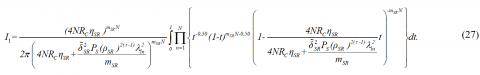${}_{2}{{F}_{1}}(a,b;c;x)=\frac{\left| \!{\overline {\, c \,}} \right. }{\left| \!{\overline {\, c-a \,}} \right. \times \left| \!{\overline {\, a \,}} \right. }\int\limits_{0}^{1}{{{t}^{b-1}}}{{(1-t)}^{c-b-1}}{{(1-tx)}^{-a}}dt.$  (28)

Comparing the above definition of the Gauss Hypergeometric function with the expression of I1 the parameters can be obtained as,

$a={{m}_{SR}}{{N}^{2}},\,\,b=0.50,\,\,c=1+{{m}_{SR}}{{N}^{2}}$ (29)

and

$x=\frac{4{{N}^{2}}{{R}_{C}}{{\eta }_{SR}}}{4{{N}^{2}}{{R}_{C}}{{\eta }_{SR}}+\frac{b\tilde{\delta }_{SR}^{2}{{P}_{S}}{{({{\rho }_{SR}})}^{2(\tau -1)}}{{\lambda }^{2}}}{{{m}_{SR}}}}.$  (30)

Using the identity given in (28) into (27), the I1  can be expressed in the form of Gauss Hypergeometric function, as given in (31).

## 31.png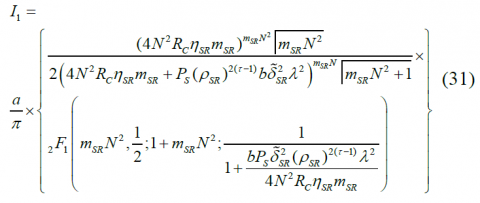I2  can be evaluated in terms of the Appell hypergeometric function of two variables:

Like the case of I1 , to solve I2 , let us change the variable by substituting ${{\sin }^{2}}\theta =t/2.$ Further manipulations of this substitution leads, $2\sin \theta \cos \theta d\theta =dt/2,$

$d\theta =\frac{dt}{4\sqrt{\frac{t}{2}}\sqrt{1-\frac{t}{2}}},$

Also, the limits of integral would change from 0 to 1. Therefore, I2  can now be given as,

\begin{align} & {{I}_{2}}=\frac{c}{\pi }{{\int\limits_{0}^{1}{\left( \frac{{{m}_{SR}}{{N}^{2}}}{{{m}_{SR}}{{N}^{2}}+\frac{b\tilde{\delta }_{SR}^{2}{{P}_{S}}{{({{\rho }_{SR}})}^{2(\tau -1)}}{{\lambda }^{2}}}{2{{R}_{C}}{{\eta }_{SR}}t}} \right)}}^{{{m}_{SR}}{{N}^{2}}}}\frac{dt}{4\sqrt{\frac{t}{2}}\sqrt{1-\frac{t}{2}}} \\ & \,\,\,\,\,\,\,\,=\frac{c}{\pi }\int\limits_{0}^{1}{{{\left( 1+\frac{b\tilde{\delta }_{SR}^{2}{{P}_{S}}{{({{\rho }_{SR}})}^{2(\tau -1)}}{{\lambda }^{2}}}{2{{R}_{C}}{{\eta }_{SR}}{{m}_{SR}}{{N}^{2}}t} \right)}^{-{{m}_{SR}}{{N}^{2}}}}}\frac{dt}{4\sqrt{\frac{t}{2}}\sqrt{1-\frac{t}{2}}} \\ & \,\,\,\,\,\,\,=\frac{c}{2\sqrt{2\pi }}\int\limits_{0}^{1}{{{t}^{{{m}_{SR}}{{N}^{2}}-1/2}}{{\left( t+\frac{b\tilde{\delta }_{SR}^{2}{{P}_{S}}{{({{\rho }_{SR}})}^{2(\tau -1)}}{{\lambda }^{2}}}{{{m}_{SR}}{{N}^{2}}2{{R}_{C}}{{\eta }_{SR}}} \right)}^{-{{m}_{SR}}{{N}^{2}}}}}\frac{dt}{\sqrt{1-\frac{t}{2}}}. \\ \end{align}   (32)

The expression above is compared with Appell hypergeometric function of two variables x' and y', defined as ,

\begin{align} & F_{A}^{(1)}({{\chi }_{1}};{{\iota }_{1}},{{{{\iota }'}}_{1}};{\gamma }'';{x}',{y}')= \\ & \frac{\Gamma ({\gamma }'')}{\Gamma ({{\chi }_{1}})\Gamma ({\gamma }''-{{\chi }_{1}})} \\ & \int\limits_{0}^{1}{{{\Theta }^{{{\chi }_{1}}-1}}{{\left( 1-\Theta \right)}^{{\gamma }''-{{\chi }_{1}}-1}}{{\left( 1-\Theta {x}' \right)}^{-{{\iota }_{1}}}}}{{\left( 1-\Theta {y}' \right)}^{-{{{{\iota }'}}_{1}}}}d\Theta \\ \end{align} (33)

Thus, comparing the integral I2  with the above definition of Appell hypergeometric function various parameters can be obtained as ,

${{\chi }_{1}}={{m}_{SR}}{{N}^{2}}+\frac{1}{2},{{\iota }_{1}}=\frac{1}{2},{{{\iota }'}_{1}}={{m}_{SR}}{{N}^{2}},{\gamma }''={{m}_{SR}}{{N}^{2}}+\frac{3}{2},{x}'=\frac{1}{2}$ and ${y}'=\frac{-{{m}_{SR}}{{N}^{2}}2{{R}_{C}}{{\eta }_{SR}}t}{b\tilde{\delta }_{SR}^{2}{{P}_{S}}{{({{\rho }_{SR}})}^{2(\tau -1)}}{{\lambda }^{2}}}.$

\begin{align} & {{I}_{2}}=\frac{c}{2\sqrt{2\pi }}{{\left( \frac{b\tilde{\delta }_{SR}^{2}{{P}_{S}}{{({{\rho }_{SR}})}^{2(\tau -1)}}{{\lambda }^{2}}}{2{{R}_{C}}{{\eta }_{SR}}{{m}_{SR}}{{N}^{2}}} \right)}^{{{m}_{SR}}{{N}^{2}}}}\frac{\Gamma ({{m}_{SR}}{{N}^{2}}+\frac{1}{2})}{\Gamma ({{m}_{SR}}{{N}^{2}}+\frac{3}{2})}\times \\ & \,\,\,\,\,\,\,\,\,\,F_{A}^{(1)}\left( {{m}_{SR}}{{N}^{2}}+\frac{1}{2};\frac{1}{2},{{m}_{SR}}{{N}^{2}};{{m}_{SR}}{{N}^{2}}+\frac{3}{2};\frac{1}{2};\frac{-{{m}_{SR}}{{N}^{2}}}{\frac{b\tilde{\delta }_{SR}^{2}{{P}_{S}}{{({{\rho }_{SR}})}^{2(\tau -1)}}{{\lambda }^{2}}}{2{{R}_{C}}{{\eta }_{SR}}}} \right) \\ \end{align} (34)

Finally we can obtain $P_{E}^{S\to R}\left( {{\text{X}}_{S}}[\tau ]\to {{\text{X}}_{l}}[\tau ] \right)$ by substituting I2 and I1 in (20). In general, when there are more than two MIMO STBC code-words, the total PEP for the decoding at relay node corresponding to the transmission of the ${{\tau }^{th}}$ MIMO STBC code-word is upper bounded by the union bound over all possible erroneous MIMO STBC code-words [9-12],

$P_{E}^{S\to R}[\tau ]\le \sum\limits_{\begin{smallmatrix} {{\text{X}}_{S}}[\tau ]\in \text{C}, \\ {{\text{X}}_{S}}[\tau ]\ne {{\text{X}}_{l}}[\tau ] \end{smallmatrix}}{P_{E}^{S\to R}\left( {{\text{X}}_{S}}[\tau ]\to {{\text{X}}_{l}}[\tau ] \right)}.$ (35)

Following a similar approach, the average PEP of the confusion event ${{X}_{S}}[\tau ]\to {{X}_{i}}[\tau ],$ corresponding to the direct SD link for the ${{\tau }^{th}}$ MIMO STBC block can be expressed as,

## 36.png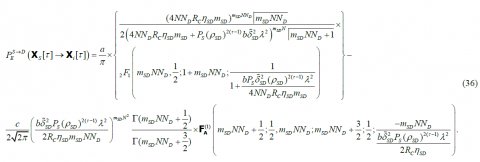where, $\tilde{\delta }_{SD}^{2}=\delta _{SD}^{2}+\sigma _{\in SD}^{2}.$ The conditional PEP expression for the error event ${{X}_{S}}[\tau ]\to {{X}_{i}}[\tau ],$ at the destination node, when the relay node successfully decodes all the symbols received from the source node during the broadcast phase, conditioned on the SD and RD channel gains $\tilde{g}_{\tilde{l},n}^{sd}(1)={{\left| {{{\tilde{\ell }}}^{sd}}_{\tilde{l},n}(1) \right|}^{2}}$ and $\tilde{g}_{l,n}^{rd}(1)={{\left| {{{\tilde{\ell }}}^{rd}}_{l,n}(1) \right|}^{2}},$ respectively, is given below,

\begin{align} & P_{E}^{SRD}\left( \left. {{X}_{S}}[\tau ]\to {{X}_{i}}[\tau ] \right|{{{\tilde{\mathbb{N}}}}_{SD}},{{{\tilde{\mathbb{N}}}}_{RD}} \right) \\ & =a\text{Q}\left( \sqrt{b\left\{ \frac{{{P}_{S}}\rho _{SD}^{2(\tau -1)}\sum\limits_{n=1}^{N}{\lambda _{in}^{2}\sum\limits_{\tilde{l}=1}^{{{N}_{D}}}{{{\left| {{{\tilde{\ell }}}^{sd}}_{\tilde{l},n}(1) \right|}^{2}}}}}{2N{{R}_{C}}\eta {}_{SD}}+\frac{{{P}_{R}}{{({{\rho }_{RD}})}^{2(\tau -1)}}\sum\limits_{n=1}^{N}{\lambda _{in}^{2}\sum\limits_{l=1}^{{{N}_{D}}}{{{\left| {{{\tilde{\ell }}}^{rd}}_{l,n}(1) \right|}^{2}}}}}{2N{{R}_{C}}\eta {}_{RD}} \right\}} \right)-c{{\text{Q}}^{2}}\left( \sqrt{b\left\{ \frac{{{P}_{S}}\rho _{SD}^{2(\tau -1)}\sum\limits_{n=1}^{N}{\lambda _{in}^{2}\sum\limits_{\tilde{l}=1}^{{{N}_{D}}}{{{\left| {{{\tilde{\ell }}}^{sd}}_{\tilde{l},n}(1) \right|}^{2}}}}}{2N{{R}_{C}}\eta {}_{SD}}+\frac{{{P}_{R}}{{({{\rho }_{RD}})}^{2(\tau -1)}}\sum\limits_{n=1}^{N}{\lambda _{in}^{2}\sum\limits_{l=1}^{{{N}_{D}}}{{{\left| {{{\tilde{\ell }}}^{rd}}_{l,n}(1) \right|}^{2}}}}}{2N{{R}_{C}}\eta {}_{RD}} \right\}} \right). \\ \end{align} (37)

where, $\tilde{\ell }_{\tilde{l},n}^{sd}(1),\tilde{\ell }_{l,n}^{rd}(1)$ are the complex Nakagami-m channel coefficients of the resulting SD and RD channel matrices ${{\tilde{\mathbb{N}}}_{SD}}={{\hat{\mathbb{N}}}_{SD}}{{U}_{i}}$ and ${{\tilde{\mathbb{N}}}_{RD}}={{\hat{\mathbb{N}}}_{RD}}{{U}_{i}},$ respectively. Applying the MGF based approach, the instantaneous PEP for the cooperation mode (SRD) fading link can be expressed as,

\begin{align} & \,P_{E}^{SRD}\left( {{\text{X}}_{S}}[\tau ]\to {{\text{X}}_{l}}[\tau ] \right)= \\ & \,\,\,\,\,\,\,\,\frac{a}{\pi }\int\limits_{0}^{\pi /2}{{{M}_{{{\gamma }_{SRD}}}}\left( \frac{b}{2{{\sin }^{2}}\theta } \right)d\theta }\,\,-\frac{c}{\pi }\int\limits_{0}^{\pi /4}{{{M}_{{{\gamma }_{SRD}}}}\left( \frac{b}{2{{\sin }^{2}}\theta } \right)d\theta }\,. \\ \end{align}

where,

\begin{align} & {{\gamma }_{SRD}} \\ & =b\left\{ \frac{{{P}_{S}}\rho _{SD}^{2(\tau -1)}\sum\limits_{n=1}^{N}{\lambda _{in}^{2}\sum\limits_{\tilde{l}=1}^{{{N}_{D}}}{{{\left| {{{\tilde{\ell }}}^{sd}}_{\tilde{l},n}(1) \right|}^{2}}}}}{4N{{R}_{C}}\eta {}_{SD}{{\sin }^{2}}\theta }+\frac{{{P}_{R}}{{({{\rho }_{RD}})}^{2(\tau -1)}}\sum\limits_{n=1}^{N}{\lambda _{in}^{2}\sum\limits_{l=1}^{{{N}_{D}}}{{{\left| {{{\tilde{\ell }}}^{rd}}_{l,n}(1) \right|}^{2}}}}}{4N{{R}_{C}}\eta {}_{RD}{{\sin }^{2}}\theta } \right\} \\ \end{align}

denotes the instantaneous SNR of the SRD fading link. Also ${{M}_{{{\gamma }_{SRD}}}}\left( s \right)$ denotes the MGF of the SRD fading link, can be expressed as,

\begin{align} & {{M}_{{{\gamma }_{SRD}}}}\left( s \right)={{\left( \frac{{{m}_{SD}}N{{N}_{D}}}{{{m}_{SD}}N{{N}_{D}}+s\frac{\tilde{\delta }_{SD}^{2}{{P}_{S}}{{({{\rho }_{SD}})}^{2(\tau -1)}}{{\lambda }^{2}}}{2{{R}_{C}}{{\eta }_{SD}}}} \right)}^{{{m}_{SD}}N{{N}_{D}}}} \\ & \,\,\,\,\,\,\,\,\,\,\,\,\,\,\,\,\,\,\,\,\times {{\left( \frac{{{m}_{RD}}N{{N}_{D}}}{{{m}_{RD}}N{{N}_{D}}+s\frac{\tilde{\delta }_{RD}^{2}{{P}_{R}}{{({{\rho }_{RD}})}^{2(\tau -1)}}{{\lambda }^{2}}}{2{{R}_{C}}{{\eta }_{RD}}}} \right)}^{{{m}_{RD}}N{{N}_{D}}}}. \\ \end{align} (38)

where, $\tilde{\delta }_{RD}^{2}=\delta _{RD}^{2}+\sigma _{\in RD}^{2}.$ Following the similar approach used in deriving the simplified expression of $P_{E}^{S\to R}\left( {{X}_{S}}[\tau ]\to {{X}_{l}}[\tau ] \right)$ & $P_{E}^{S\to D}\left( {{X}_{S}}[\tau ]\to {{X}_{i}}[\tau ] \right)$, the average PEP for the cooperation mode, $P_{E}^{SRD}\left( {{X}_{S}}[\tau ]\to {{X}_{i}}[\tau ] \right)$ can be expressed, as given in (39). The end-to-end error probability expression for the erroneous event ${{X}_{S}}[\tau ]\to {{X}_{i}}[\tau ],$ corresponding to MIMO STBC code-word transmission can be written as,

## 39.png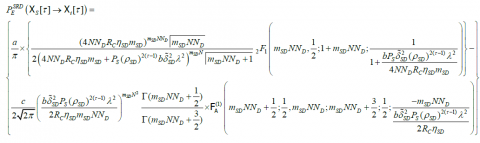\times \left\{ \begin{align} & \frac{a}{\pi }\times \left\{ \frac{{{(4N{{N}_{D}}{{R}_{C}}{{\eta }_{RD}}{{m}_{RD}})}^{{{m}_{RD}}N{{N}_{D}}}}\left| \!{\overline {\, {{m}_{RD}}N{{N}_{D}} \,}} \right. }{2{{\left( 4N{{N}_{D}}{{R}_{C}}{{\eta }_{RD}}{{m}_{SD}}+{{P}_{S}}{{({{\rho }_{RD}})}^{2(\tau -1)}}b\tilde{\delta }_{RD}^{2}{{\lambda }^{2}} \right)}^{{{m}_{SD}}N}}\left| \!{\overline {\, {{m}_{RD}}N{{N}_{D}}+1 \,}} \right. }{}_{2}{{F}_{1}}\left( {{m}_{RD}}N{{N}_{D}},\frac{1}{2};1+{{m}_{RD}}N{{N}_{D}};\frac{1}{1+\frac{b{{P}_{S}}\tilde{\delta }_{RD}^{2}{{({{\rho }_{RD}})}^{2(\tau -1)}}{{\lambda }^{2}}}{4N{{N}_{D}}{{R}_{C}}{{\eta }_{RD}}{{m}_{RD}}}} \right) \right\} \\ & -\frac{c}{2\sqrt{2\pi }}{{\left( \frac{b\tilde{\delta }_{RD}^{2}{{P}_{R}}{{({{\rho }_{RD}})}^{2(\tau -1)}}{{\lambda }^{2}}}{2{{R}_{C}}{{\eta }_{RD}}{{m}_{RD}}N{{N}_{D}}} \right)}^{{{m}_{SD}}{{N}^{2}}}}\frac{\Gamma ({{m}_{RD}}N{{N}_{D}}+\frac{1}{2})}{\Gamma ({{m}_{RD}}N{{N}_{D}}+\frac{3}{2})}\times \text{F}_{\text{A}}^{(1)}\left( {{m}_{RD}}N{{N}_{D}}+\frac{1}{2};\frac{1}{2},{{m}_{RD}}N{{N}_{D}};{{m}_{RD}}N{{N}_{D}}+\frac{3}{2};\frac{1}{2};\frac{-{{m}_{RD}}N{{N}_{D}}}{\frac{b\tilde{\delta }_{RD}^{2}{{P}_{S}}{{({{\rho }_{RD}})}^{2(\tau -1)}}{{\lambda }^{2}}}{2{{R}_{C}}{{\eta }_{RD}}}} \right) \\\end{align} \right\}  (39)

\begin{align} & {{P}^{E}}[{{X}_{S}}[\tau ]\to {{X}_{i}}[\tau ]]=\,\,\underbrace{P_{E}^{S\to D}\left[ {{X}_{S}}[\tau ]\to {{X}_{i}}[\tau ] \right]}_{\begin{smallmatrix} \text{Direct}\,\,\text{SD}\,\,\text{Transmission} \\ \,\text{Error}\,\text{Probability}\end{smallmatrix}}\times \\ & \underbrace{P_{E}^{S\to R}}_{\begin{smallmatrix} \text{Error}\,\text{Probability}\,\text{when}\,\text{relay} \\ \,\text{decodes}\,\,\,\text{incorrectly}\end{smallmatrix}}+\underbrace{(1-P_{E}^{S\to R})}_{\begin{smallmatrix} \text{Probability}\,\text{when}\,\text{relay}\, \\ \text{decodes}\,\,\,\text{correctly}\end{smallmatrix}}\times \\ & \underbrace{P_{E}^{SRD}\left( {{X}_{S}}[\tau ]\to {{X}_{i}}[\tau ] \right)}_{\begin{smallmatrix} \text{SRD(Cooperation}\,\text{Mode}\,\text{Transmission)}\,\, \\ \text{Transmission}\,\text{Error Probability}\end{smallmatrix}}. \\\end{align}    (40)

Substituting (20), (36) and (39) into (40) and employing the fact that $1-P_{E}^{S\to R}\approx 1$ at high SNR in (40), yields the expression for the average end-to-end PEP ${{P}^{E}}[{{X}_{S}}[\tau ]\to {{X}_{i}}[\tau ]]$ corresponds to the erroneous event ${{X}_{S}}[\tau ]\to {{X}_{i}}[\tau ]$ when ${{X}_{S}}[\tau ]$ confused for the ${{X}_{i}}[\tau ],$ as given  in (41). Using the end-to-end error probability expression given in (41), the error bound for the ML decoding (optimum decoding) at the destination node corresponding to ${{\tau }^{th}}$ MIMO-STBC code-word block can now be readily obtained by considering the union bound over all the PEP terms corresponding to the ${{X}_{S}}[\tau ]\to {{X}_{i}}[\tau ]$ over-all the possible code-words ${{\text{X}}_{S}}[\tau ]\in \text{C}\text{.}$ Finally, the per-block average PEP bound at the destination can be expressed, as given in (42).

## 411.png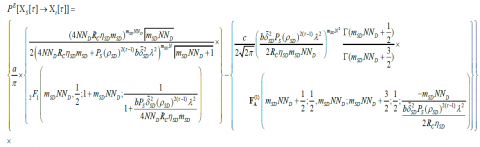## 412.png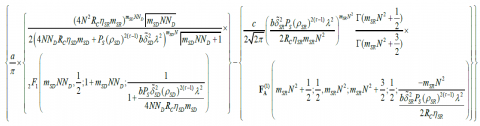\,+\left\{ \begin{align} & \frac{a}{\pi }\times \left\{ \frac{{{(4N{{N}_{D}}{{R}_{C}}{{\eta }_{SD}}{{m}_{SD}})}^{{{m}_{SD}}N{{N}_{D}}}}\left| \!{\overline {\, {{m}_{SD}}N{{N}_{D}} \,}} \right. }{2{{\left( 4N{{N}_{D}}{{R}_{C}}{{\eta }_{SD}}{{m}_{SD}}+{{P}_{S}}{{({{\rho }_{SD}})}^{2(\tau -1)}}b\tilde{\delta }_{SD}^{2}{{\lambda }^{2}} \right)}^{{{m}_{SD}}N}}\left| \!{\overline {\, {{m}_{SD}}N{{N}_{D}}+1 \,}} \right. }{}_{2}{{F}_{1}}\left( {{m}_{SD}}N{{N}_{D}},\frac{1}{2};1+{{m}_{SD}}N{{N}_{D}};\frac{1}{1+\frac{b{{P}_{S}}\tilde{\delta }_{SD}^{2}{{({{\rho }_{SD}})}^{2(\tau -1)}}{{\lambda }^{2}}}{4N{{N}_{D}}{{R}_{C}}{{\eta }_{SD}}{{m}_{SD}}}} \right) \right\}- \\ & \frac{c}{2\sqrt{2\pi }}{{\left( \frac{b\tilde{\delta }_{SD}^{2}{{P}_{S}}{{({{\rho }_{SD}})}^{2(\tau -1)}}{{\lambda }^{2}}}{2{{R}_{C}}{{\eta }_{SD}}{{m}_{SD}}N{{N}_{D}}} \right)}^{{{m}_{SD}}{{N}^{2}}}}\frac{\Gamma ({{m}_{SD}}N{{N}_{D}}+\frac{1}{2})}{\Gamma ({{m}_{SD}}N{{N}_{D}}+\frac{3}{2})}\times \,\text{F}_{\text{A}}^{(1)}\left( {{m}_{SD}}N{{N}_{D}}+\frac{1}{2};\frac{1}{2},{{m}_{SD}}N{{N}_{D}};{{m}_{SD}}N{{N}_{D}}+\frac{3}{2};\frac{1}{2};\frac{-{{m}_{SD}}N{{N}_{D}}}{\frac{b\tilde{\delta }_{SD}^{2}{{P}_{S}}{{({{\rho }_{SD}})}^{2(\tau -1)}}{{\lambda }^{2}}}{2{{R}_{C}}{{\eta }_{SD}}}} \right) \\\end{align} \right\}

\,\times \left\{ \begin{align} & \frac{a}{\pi }\times \left\{ \frac{{{(4N{{N}_{D}}{{R}_{C}}{{\eta }_{RD}}{{m}_{RD}})}^{{{m}_{RD}}N{{N}_{D}}}}\left| \!{\overline {\, {{m}_{RD}}N{{N}_{D}} \,}} \right. }{2{{\left( 4N{{N}_{D}}{{R}_{C}}{{\eta }_{RD}}{{m}_{SD}}+{{P}_{S}}{{({{\rho }_{RD}})}^{2(\tau -1)}}b\tilde{\delta }_{RD}^{2}{{\lambda }^{2}} \right)}^{{{m}_{SD}}N}}\left| \!{\overline {\, {{m}_{RD}}N{{N}_{D}}+1 \,}} \right. }{}_{2}{{F}_{1}}\left( {{m}_{RD}}N{{N}_{D}},\frac{1}{2};1+{{m}_{RD}}N{{N}_{D}};\frac{1}{1+\frac{b{{P}_{S}}\tilde{\delta }_{RD}^{2}{{({{\rho }_{RD}})}^{2(\tau -1)}}{{\lambda }^{2}}}{4N{{N}_{D}}{{R}_{C}}{{\eta }_{RD}}{{m}_{RD}}}} \right) \right\} \\ & -\frac{c}{2\sqrt{2\pi }}{{\left( \frac{b\tilde{\delta }_{RD}^{2}{{P}_{R}}{{({{\rho }_{RD}})}^{2(\tau -1)}}{{\lambda }^{2}}}{2{{R}_{C}}{{\eta }_{RD}}{{m}_{RD}}N{{N}_{D}}} \right)}^{{{m}_{SD}}{{N}^{2}}}}\frac{\Gamma ({{m}_{RD}}N{{N}_{D}}+\frac{1}{2})}{\Gamma ({{m}_{RD}}N{{N}_{D}}+\frac{3}{2})}\times \text{F}_{\text{A}}^{(1)}\left( {{m}_{RD}}N{{N}_{D}}+\frac{1}{2};\frac{1}{2},{{m}_{RD}}N{{N}_{D}};{{m}_{RD}}N{{N}_{D}}+\frac{3}{2};\frac{1}{2};\frac{-{{m}_{RD}}N{{N}_{D}}}{\frac{b\tilde{\delta }_{RD}^{2}{{P}_{S}}{{({{\rho }_{RD}})}^{2(\tau -1)}}{{\lambda }^{2}}}{2{{R}_{C}}{{\eta }_{RD}}}} \right) \\ \end{align} \right\}.  (41)

## 42.png3.2 Asymptotic error floor analysis

Now, in order to theoretically support our claims about the performance degradation due to imperfect-CSI and mobile nodes, it is useful to obtain the asymptotic error floors for the single relay S-DF protocol by ignoring N0 in (10), (14) and (15) for single relay scenario, as expressed below [16-17],

${{\tilde{\eta }}_{SD}}=\rho _{SD}^{2(\tau -1)}\sigma _{_{{{\in }_{SD}}}}^{2}+\left( 1-\rho _{SD}^{2(\tau -1)} \right)\sigma _{_{{{e}_{SD}}}}^{2},$   (43)

${{\tilde{\eta }}_{SR}}={{({{\rho }_{SR}})}^{2(\tau -1)}}{{({{\sigma }_{\in SR}})}^{2}}+\left( 1-{{({{\rho }_{SR}})}^{2(\tau -1)}} \right){{({{\sigma }_{eSR}})}^{2}},$  (44)

${{\tilde{\eta }}_{RD}}={{({{\rho }_{RD}})}^{2(\tau -1)}}{{({{\sigma }_{\in RD}})}^{2}}+\left( 1-{{({{\rho }_{RD}})}^{2(\tau -1)}} \right){{({{\sigma }_{eRD}})}^{2}},$     (45)

Using ${{\tilde{\eta }}_{SD}},{{\tilde{\eta }}_{SR}}$ and ${{\tilde{\eta }}_{RD}}$ in (42), one can readily obtain the asymptotic error floor for per block average PEP, as given in (46).

Various conditions arise due to nodes mobility:

When all nodes are static and perfect CSI condition

According to Jake’s autocorrelation model , when all of the nodes are static, i.e., the relative speed between any two communicating nodes of them is zero, the correlation parameters  ${{\rho }_{SR}},{{\rho }_{SD}}$ and ${{\rho }_{RD}}$ reduce to 1. By considering this condition, the asymptotic limit for ${{P}^{E}}$ is still given by (46), but with the following modified parameters: ${{\tilde{\eta }}_{SD}}=\sigma _{_{{{\in }_{SD}}}}^{2},{{\tilde{\eta }}_{SR}}=\sigma _{\in SR}^{2},$ and ${{\tilde{\eta }}_{RD}}=\sigma _{\in RD}^{2}.$ Furthermore, if the estimation processes throughout the network are perfect, i.e., $\sigma _{_{{{\in }_{SD}}}}^{2}=\sigma _{\in SR}^{2}=\sigma _{\in RD}^{2}=0$, the value of ${{\tilde{\eta }}_{SD}},{{\tilde{\eta }}_{SR}}$ and ${{\tilde{\eta }}_{RD}}$ reduces to zero which reduces (46) to zero, i.e., the asymptotic error floor in (46), as expected, vanish, i.e., impact of node mobility is removed.

When source and destination nodes are static and relay node is mobile

Corollary 1: In DH single relay S-DF cooperative communication networks with perfect CSI, even though the relay is in motion, the system performance does not experience asymptotic limits if both source and destination are static.

Proof: In this scenario, ${{\rho }_{SD}}=1$ while ${{\rho }_{SR}}$ and ${{\rho }_{RD}}$ are <1. Under this condition, the asymptotic limit for PE is still given by (46), but with the following modified parameters:

${{\tilde{\eta }}_{SD}}=\sigma _{_{{{\in }_{SD}}}}^{2},\,\,{{\tilde{\eta }}_{SR}}={{({{\rho }_{SR}})}^{2(\tau -1)}}\sigma _{_{\in SR}}^{2}+\left( 1-{{({{\rho }_{SR}})}^{2(\tau -1)}} \right)\sigma _{_{eSR}}^{2},$

${{\tilde{\eta }}_{RD}}={{({{\rho }_{RD}})}^{2(\tau -1)}}\sigma _{\in RD}^{2}+\left( 1-{{({{\rho }_{RD}})}^{2(\tau -1)}} \right)\sigma _{eRD}^{2}.$

Furthermore, if the estimation processes throughout the network are perfect, i.e., $\sigma _{_{{{\in }_{SD}}}}^{2}=\sigma _{\in SR}^{2}=\sigma _{\in RD}^{2}=0$, the value of ${{\tilde{\eta }}_{SD}},{{\tilde{\eta }}_{SR}}$ and ${{\tilde{\eta }}_{RD}}$ reduces to zero which reduces (46) to zero, i.e., the asymptotic error floor in (46), as expected, vanish, i.e., impact of node mobility is removed. Despite that both scenarios (a) and (b) do not provide asymptotic limits in the case of perfect estimation, this does not mean that they provide same performance results over all the non-infinite SNR range. Later in the simulation results section, we will show that scenario (b) provides worse performance than scenario (a) due to the relay mobility.

When relay and source nodes are static and destination node is mobile

Corollary 2: In DH single relay S-DF cooperative communication networks, even though relay is static, the system performance is severely degraded by asymptotic limits if destination node is in motion.

Proof: When the destination node is in motion and the other nodes are static; ${{\rho }_{SR}}=1$ while ${{\rho }_{SD}}$ and ${{\rho }_{RD}}$ are <1. In this case, the asymptotic limit is given in (46), but with the following modified parameters:

${{\tilde{\eta }}_{SR}}=\sigma _{_{\in SR}}^{2},{{\tilde{\eta }}_{SD}}=\rho _{SD}^{2(\tau -1)}\sigma _{_{{{\in }_{SD}}}}^{2}+\left( 1-\rho _{SD}^{2(\tau -1)} \right)\sigma _{_{{{e}_{SD}}}}^{2}$

and ${{\tilde{\eta }}_{RD}}={{({{\rho }_{RD}})}^{2(\tau -1)}}\sigma _{\in RD}^{2}+\left( 1-{{({{\rho }_{RD}})}^{2(\tau -1)}} \right)\sigma _{eRD}^{2}.$

In this scenario, it is significant to note that the PEP term for the event when relay node decodes the MIMO STBC code-word correctly, i.e., $\,\underbrace{(1-P_{E}^{S\to R})}_{\begin{smallmatrix} \text{Probability}\,\text{when}\,\text{relay}\, \\ \text{decodes}\,\,\,\text{correctly} \end{smallmatrix}}$  in (40) contributes to the asymptotic error floor for the per-block average PEP.

When relay and destination nodes are static and source node is mobile

Corollary 3: In DH single relay S-DF cooperative communication networks, even though relay is static, the system performance is severely degraded by asymptotic limits if the source node is in motion.

Proof:  When the source node is in motion and the other nodes are static; ${{\rho }_{RD}}=1$ while ${{\rho }_{SD}}$ and ${{\rho }_{SR}}$ are <1. In this case, the asymptotic limit is given by (46), but with the following modified parameters:

${{\tilde{\eta }}_{RD}}={{({{\sigma }_{\in RD}})}^{2}},$  ${{\tilde{\eta }}_{SD}}=\rho _{SD}^{2(\tau -1)}\sigma _{_{{{\in }_{SD}}}}^{2}+\left( 1-\rho _{SD}^{2(\tau -1)} \right)\sigma _{_{{{e}_{SD}}}}^{2},$

and ${{\tilde{\eta }}_{SR}}={{({{\rho }_{SR}})}^{2(\tau -1)}}{{({{\sigma }_{\in SR}})}^{2}}+$$\left( 1-{{({{\rho }_{SR}})}^{2(\tau -1)}} \right){{({{\sigma }_{eSR}})}^{2}}.$

In this scenario, it is significant to note that the PEP term for the event when relay node decodes the MIMO STBC code-word incorrectly, i.e., $\underbrace{P_{E}^{S\to R}}_{\begin{smallmatrix} \text{Error}\,\text{Probability}\,\text{when}\,\text{relay} \\ \,\text{decodes}\,\,\,\text{incorrectly}\end{smallmatrix}}$ in (40) contributes to the asymptotic error floor for the per-block average PEP.

## 46.png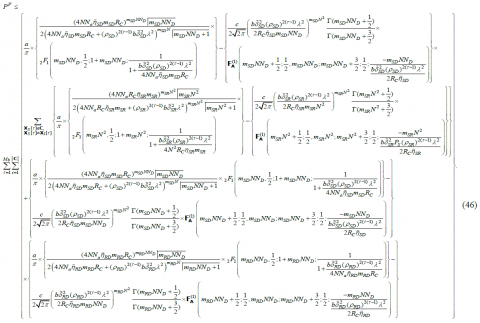3.3 DO analysis and optimal power allocation

3.3.1 DO Analysis

In this sub-section we demonstrate the DO analysis and will develop a framework to evaluate the optimal source-relay power allocation factors, which will further enhance the end-to-end error performance of the relaying network. At high SNR, since the union bound is tight, the source and relay SNRs, ${{P}_{S}}/{{N}_{0}},{{P}_{R}}/{{N}_{0}}\to \infty$. We consider that each receiving terminal has perfect channel estimation, i.e., $\sigma _{\in SD}^{2}=\sigma _{\in SR}^{2}=\sigma _{\in RD}^{2}=0$ and nodes are static, i.e., $\sigma _{eSD}^{2}=\sigma _{eSR}^{2}=\sigma _{eRD}^{2}=0$. Applying the above mentioned conditions in (41) and at high SNR, considering the dominating terms corresponding to $m,n=0,1$ in the identity ${}_{2}{{F}_{1}}(a,b;c;z)=\sum\limits_{n=0}^{K}{\frac{{{(a)}_{n}}{{(b)}_{n}}{{z}^{n}}}{{{(c)}_{n}}n!}}$ for the Gauss Hypergeometric function and Appell hypergeometric function of two variables ,

\begin{align} & \text{F}_{\text{A}}^{(1)}({{\chi }_{1}},{{\iota }_{1}},{{{{\iota }'}}_{1}},{\gamma }'';{x}',{y}')= \\ & \sum\limits_{m,n=0}^{\infty }{\frac{{{\left( {{\chi }_{1}} \right)}_{m+n}}{{\left( {{\iota }_{1}} \right)}_{m}}{{\left( {{{{\iota }'}}_{1}} \right)}_{n}}}{{{\left( {{\gamma }''} \right)}_{m+n}}\Gamma \left( m+1 \right)\Gamma \left( n+1 \right)}}{{\left( {{x}'} \right)}^{m}}{{\left( {{y}'} \right)}^{n}}, \\\end{align}

where, ${{(x)}_{n}}$ is the Pochhammer symbols , one can simplify the expression in (41), as given in (47).

## 47.png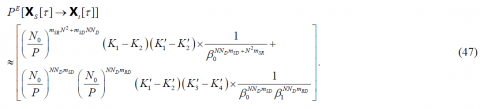where, ${{\beta }_{0}}$ and ${{\beta }_{1}}$ are the optimal source-relay power factors,

\begin{align} & {{\beta }_{0}}={{P}_{S}}/P,\,\,{{\beta }_{1}}= \\ & {{P}_{R}}/P,\,\,{{K}_{1}}=\frac{a}{\pi }{{\left( \frac{2{{R}_{C}}{{m}_{SR}}{{N}^{2}}}{b\delta _{SR}^{2}} \right)}^{{{m}_{SR}}{{N}^{2}}}}\int\limits_{0}^{\pi /2}{{{\left( {{\sin }^{2}}\theta \right)}^{{{m}_{SR}}{{N}^{2}}}}}d\theta , \\\end{align}

${{K}_{2}}=\frac{c}{\pi }{{\left( \frac{2{{R}_{C}}{{m}_{SR}}{{N}^{2}}}{b\delta _{SR}^{2}} \right)}^{{{m}_{SR}}{{N}^{2}}}}\int\limits_{0}^{\pi /4}{{{\left( {{\sin }^{2}}\theta \right)}^{{{m}_{SR}}{{N}^{2}}}}},$

\begin{align} & {{{{K}'}}_{1}}=\frac{a}{\pi }{{\left( \frac{2{{R}_{C}}{{m}_{SD}}N{{N}_{D}}}{b\delta _{SD}^{2}} \right)}^{{{m}_{SD}}N{{N}_{D}}}}\int\limits_{0}^{\pi /2}{{{\left( {{\sin }^{2}}\theta \right)}^{{{m}_{SD}}N{{N}_{D}}}}}d\theta \\ & {{{{K}'}}_{2}}=\frac{c}{\pi }{{\left( \frac{2{{R}_{C}}{{m}_{SD}}N{{N}_{D}}}{b\delta _{SD}^{2}} \right)}^{{{m}_{SD}}N{{N}_{D}}}}\int\limits_{0}^{\pi /4}{{{\left( {{\sin }^{2}}\theta \right)}^{{{m}_{SD}}N{{N}_{D}}}}}d\theta \\\end{align}

\begin{align} & {{{{K}'}}_{3}}=\frac{a}{\pi }{{\left( \frac{2{{R}_{C}}{{m}_{RD}}N{{N}_{D}}}{b\delta _{RD}^{2}} \right)}^{{{m}_{RD}}N{{N}_{D}}}}\int\limits_{0}^{\pi /2}{{{\left( {{\sin }^{2}}\theta \right)}^{{{m}_{RD}}N{{N}_{D}}}}}d\theta \\ & {{{{K}'}}_{4}}=\frac{c}{\pi }{{\left( \frac{2{{R}_{C}}{{m}_{RD}}N{{N}_{D}}}{b\delta _{RD}^{2}} \right)}^{{{m}_{RD}}N{{N}_{D}}}}\int\limits_{0}^{\pi /4}{{{\left( {{\sin }^{2}}\theta \right)}^{{{m}_{RD}}N{{N}_{D}}}}}d\theta \\\end{align}

Using the PEP expression expressed in (47) for the error event ${{X}_{S}}[\tau ]\to {{X}_{i}}[\tau ]$, the asymptotic PEP approximation of the above system can be obtained at high SNR employing the union bound, as given in (48). Asymptotically tight PEP expression expressed in (48) can be further simplified as,

${{P}^{E}}\le {{\Re }_{1}}{{({{N}_{0}}/P)}^{N{{N}_{D}}{{m}_{SD}}+{{N}^{2}}{{m}_{SR}}}}+{{\Re }_{2}}{{({{N}_{0}}/P)}^{N{{N}_{D}}{{m}_{SD}}+N{{N}_{D}}{{m}_{RD}}}},$  (48)

where, the terms ${{\Re }_{1}}$ and ${{\Re }_{2}}$ are appropriately defined constant terms.

Depending upon the various values of the product of the DO and shape parameter of the fading links, various cases arise,

Case1: When the product of the DO and shape parameter of the RD fading link is greater than the product of the DO and shape parameter of the SR fading link, i.e., $N{{N}_{D}}{{m}_{RD}}>{{N}^{2}}{{m}_{SR}}.$ Then in high SNR regimes, the first term is the dominant term in PEP expression, PEP can be expressed as,

${{P}^{E}}\le {{\Re }_{1}}{{({{N}_{0}}/P)}^{N{{N}_{D}}{{m}_{SD}}+{{N}^{2}}{{m}_{SR}}}}.$

So, DO can be derived as,

$DO=-\underbrace{\lim }_{P/{{N}_{0}}\to \infty }\frac{\log ({{P}^{E}})}{\log (P/{{N}_{0}})}=N{{N}_{D}}{{m}_{SD}}+{{N}^{2}}{{m}_{SR}}.$   (49)

Case 2: When the product of the DO and shape parameter of the SR fading link is greater than the product of the DO and shape parameter of the RD fading link, i.e., $N{{N}_{D}}{{m}_{RD}}<{{N}^{2}}{{m}_{SR}}.$ Then in high SNR regimes, the second term is the dominant term in PEP expression, PEP can be written as,

${{P}^{E}}\le {{\Re }_{2}}{{({{N}_{0}}/P)}^{N{{N}_{D}}{{m}_{SD}}+N{{N}_{D}}{{m}_{RD}}}}.$ (50)

So, DO can be derived as,

$DO=-\underbrace{\lim }_{P/{{N}_{0}}\to \infty }\frac{\log ({{P}^{E}})}{\log (P/{{N}_{0}})}=N{{N}_{D}}{{m}_{SD}}+N{{N}_{D}}{{m}_{RD}}.$ (51)

Also, for the general case, when the product of the DO and shape parameter of the SR fading link is equal to the product of the DO and shape parameter of the RD fading link, i.e., $N{{N}_{D}}{{m}_{RD}}={{N}^{2}}{{m}_{SR}}.$ Then in high SNR regimes, the DO can be expressed as,

\begin{align} & DO=\min (N{{N}_{D}}{{m}_{SD}}+{{N}^{2}}{{m}_{SR}},N{{N}_{D}}{{m}_{SD}}+N{{N}_{D}}{{m}_{RD}})= \\ & \,\,\,\,\,\,\,\,\,\,\,\,\,N{{N}_{D}}{{m}_{SD}}+N\min (N{{m}_{SR}},{{N}_{D}}{{m}_{RD}}). \\ \end{align}  (52)

3.3.2 Optimal power allocation

The expression (47) can be modeled as a CO problem for deriving the optimal source-relay power allocation factors ${{\beta }_{0}}$ and ${{\beta }_{1}}$.

CO problem is modeled as,

$\underset{{{\beta }_{0}},\,\,{{\beta }_{1}}}{\mathop{\min }}\,\left\{ \frac{{{\varpi }_{1}}}{\beta _{0}^{N{{N}_{D}}{{m}_{SD}}+{{N}^{2}}{{m}_{SR}}}}+\frac{{{\varpi }_{2}}}{\beta _{0}^{N{{N}_{D}}{{m}_{SD}}}\beta _{1}^{N{{N}_{D}}{{m}_{RD}}}} \right\},$

$s.t.\,\,\,{{\beta }_{0}}+{{\beta }_{1}}\le 1,$

and,

$\,{{\beta }_{0}},{{\beta }_{1}}\ge 0.$ (53)

where, ${{\varpi }_{1}}$ and ${{\varpi }_{2}}$ are expressed below,

${{\varpi }_{1}}={{\left( \frac{{{N}_{0}}}{P} \right)}^{{{m}_{SR}}{{N}^{2}}+{{m}_{SD}}N{{N}_{D}}}}\left( {{K}_{1}}-{{K}_{2}} \right)\left( {{{{K}'}}_{1}}-{{{{K}'}}_{2}} \right),$

${{\varpi }_{2}}={{\left( \frac{{{N}_{0}}}{P} \right)}^{N{{N}_{D}}{{m}_{SD}}+N{{N}_{D}}{{m}_{RD}}}}\left( {{{{K}'}}_{1}}-{{{{K}'}}_{2}} \right)\left( {{{{K}'}}_{3}}-{{{{K}'}}_{4}} \right).$ (54)

One can see that the KKT conditions-based CO framework can be employed for deriving the optimal source-relay power allocation factors ${{\beta }_{0}}$ and ${{\beta }_{1}}$ In the mathematical CO framework, the KKT conditions are $\,{{1}^{st}}$ order necessary conditions for finding the optimal solution of nonlinear programming (NLP) , subject to the satisfaction of some regularity conditions. Allowing inequality constraints, the KKT will tend to NLP generalizes the concept of Lagrange multiplier which allows only equality constraints. Consider the following nonlinear minimization problem:

$\underset{{{\beta }_{0}},\,\,{{\beta }_{1}}}{\mathop{\min }}\,\,\,f\left( {{\beta }_{0}},{{\beta }_{1}}\, \right)=\frac{{{\varpi }_{1}}}{\beta _{0}^{N{{N}_{D}}{{m}_{SD}}+{{N}^{2}}{{m}_{SR}}}}+\frac{{{\varpi }_{2}}}{\beta _{0}^{N{{N}_{D}}{{m}_{SD}}}\beta _{1}^{N{{N}_{D}}{{m}_{RD}}}},$

$s.t.\,\,{{\beta }_{i}}\ge 0,\,\,\,\,\,\,i=0,1.$   (55)

where, ${{\varpi }_{1}}$ and ${{\varpi }_{2}}$ are given as,

${{\varpi }_{1}}={{\left( \frac{{{N}_{0}}}{P} \right)}^{{{m}_{SR}}{{N}^{2}}+{{m}_{SD}}N{{N}_{D}}}}\left( {{K}_{1}}-{{K}_{2}} \right)\left( {{{{K}'}}_{1}}-{{{{K}'}}_{2}} \right),$   (56)

${{\varpi }_{2}}={{\left( \frac{{{N}_{0}}}{P} \right)}^{N{{N}_{D}}{{m}_{SD}}}}{{\left( \frac{{{N}_{0}}}{P} \right)}^{N{{N}_{D}}{{m}_{RD}}}}\left( {{{{K}'}}_{1}}-{{{{K}'}}_{2}} \right)\left( {{{{K}'}}_{3}}-{{{{K}'}}_{4}} \right).$    (57)

By changing ${{\beta }_{i}}\ge 0$ to $-{{\beta }_{i}}\le 0$ and noting that the above CO problem is minimization problem, the Lagrangian based mathematical framework is given as,

\begin{align} & {L}'\left( {{\beta }_{0}},{{\beta }_{1}} \right)= \\ & \frac{{{\varpi }_{1}}}{\beta _{0}^{N{{N}_{D}}{{m}_{SD}}+{{N}^{2}}{{m}_{SR}}}}+\frac{{{\varpi }_{2}}}{\beta _{0}^{N{{N}_{D}}{{m}_{SD}}}\beta _{1}^{N{{N}_{D}}{{m}_{RD}}}}+{\zeta }'\left( {{\beta }_{0}}+{{\beta }_{1}}-1 \right),\,\,\,{\zeta }'>0 \\\end{align}  (58)

where, ${\zeta }'$ denotes the Lagrange multiplier. Differentiating ${L}'\left( {{\beta }_{0}},{{\beta }_{1}} \right)$ with respect to ${{\beta }_{0}}$, we get,

\begin{align} & \frac{\partial {L}'\left( {{\beta }_{0}},{{\beta }_{1}} \right)}{\partial {{\beta }_{0}}}=\frac{-{{\varpi }_{1}}\left( N{{N}_{D}}{{m}_{SD}}+{{N}^{2}}{{m}_{SR}} \right)}{\beta _{0}^{N{{N}_{D}}{{m}_{SD}}+{{N}^{2}}{{m}_{SR}}}} \\ & +\frac{-{{\varpi }_{2}}\left( N{{N}_{D}}{{m}_{SD}} \right)}{\beta _{0}^{N{{N}_{D}}{{m}_{SD}}+1}\beta _{1}^{N{{N}_{D}}{{m}_{RD}}}}+{\zeta }', \\ \end{align}  (59)

Putting (59) equal to zero, we get,

${\zeta }'=\frac{{{\varpi }_{1}}\left( N{{N}_{D}}{{m}_{SD}}+{{N}^{2}}{{m}_{SR}} \right)}{\beta _{0}^{N{{N}_{D}}{{m}_{SD}}+{{N}^{2}}{{m}_{SR}}}}+\frac{{{\varpi }_{2}}\left( N{{N}_{D}}{{m}_{SD}} \right)}{\beta _{0}^{N{{N}_{D}}{{m}_{SD}}+1}\beta _{1}^{N{{N}_{D}}{{m}_{RD}}}},$  (60)

Differentiating ${L}'\left( {{\beta }_{0}},{{\beta }_{1}} \right)$ with respect to ${{\beta }_{1}}$ and putting the resultant expression equal to zero, we get,

${\zeta }'=\frac{{{\varpi }_{2}}\left( N{{N}_{D}}{{m}_{RD}} \right)}{\beta _{0}^{N{{N}_{D}}{{m}_{SD}}}\beta _{1}^{N{{N}_{D}}{{m}_{RD}}+1}},$  (61)

Substituting the value of ${\zeta }'$ obtained in (61) into (60), we get,

${{\left( 1-{{\beta }_{0}} \right)}^{N{{N}_{D}}{{m}_{RD}}+1}}\varepsilon -{{\left( {{\beta }_{0}} \right)}^{{{N}^{2}}{{m}_{SR}}+1}}+\left( 1-{{\beta }_{0}} \right){{\left( {{\beta }_{0}} \right)}^{{{N}^{2}}{{m}_{SR}}}}=0.$   (62)

where, $\varepsilon$  is expressed in (63). Further, we can find out the solution of the quadratic expression given in the equation (62) by using standard mathematical computing software such as MATHEMATICA or MATLAB.

## 63.png4. Simulation Results

Monte Carlo (MC) simulations are conducted to confirm the accuracy of the theoretical results for the considered MIMO STBC based S-DF cooperative communication protocol over time selective Nakagami-m fading channel conditions considering imperfect CSI and node mobility conditions. Due to nodes mobility, the system's links are characterized by time-selective fading channels, which are modeled by the AR1 process, and due to incorrect CSI estimation, the estimated channel variances are assumed to be corrupted by Gaussian errors. For such a system model, an approach is proposed to derive a tight approximate expression for the system's conditional PEP. This approach is based on utilizing the AR1 model to derive exact expressions for the per block average PEP of the Alamouti decoder's decision variables, and on benefiting from the central limit theorem to approximate some of the non-Gaussian interference and noise terms. The obtained conditional PEP expression is function of both the fading channel correlation parameters and the estimation error variances, and thus, it is valid for mobile as well as static nodes for imperfect as well as perfect channel state information estimation processes. We consider S-DF relaying protocol and assume that the relay can check whether the decoding result is correct or not. Per block average PEP performance is investigated for both equal and optimal power allocation scenarios. To investigate the accuracy of the investigation for M-PSK signals, we employ M = 4 to check the M-PSK results. Figure 2. shows the PEP performance of dual phase DH relaying protocol over time selective Nakagami-m channel with imperfect CSI and node mobility for equal and optimal power allocation.  The results show that analytical results are in exact match with the simulated results at high SNR regimes and the per block average PEP performance for optimal power is better than PEP performance for equal power allocation. Figure 3. shows the per block average PEP performance of MIMO Alamouti STBC S-DF relaying protocol with QPSK modulation for various node mobility scenarios. Results show that for the network conditions when, ${{\rho }_{SR}}<1,{{\rho }_{RD}}=1,{{\rho }_{SD}}<1,$ source mobility significantly degrades the per block average PEP performance in contrast to the network condition with only relay is mobile, i.e., ${{\rho }_{SR}}<1,{{\rho }_{RD}}<1,{{\rho }_{SD}}=1.$

## 2.pngFigure 2. Per block average PEP vs. SNR in dB of MIMO Alamouti STBC S-DF relaying protocol with QPSK modulation for optimal and equal power allocation with ${{f}_{C}}=5.9GHz,{{R}_{S}}=9.6\,kbps,{{N}_{0}}=1,{{R}_{C}}=1,N={{N}_{D}}=2,\sigma _{{{\in }_{i}}}^{2}=0.01,$  $\sigma _{{{e}_{i}}}^{2}=0.01;i\in \left\{ SD,SR,RD \right\},{{v}_{p}}=50mph,\delta _{SD}^{2}=2,\delta _{SR}^{2}=2,\delta _{RD}^{2}=2$ and optimal power allocation factors are ${{\beta }_{0}}=0.3843,$ ${{\beta }_{1}}=0.3078$ and ${{\beta }_{2}}=0.3078,{{M}_{b}}=5,$ respectively

## 3.pngFigure 3. Per block average PEP vs. SNR in dB of MIMO Alamouti STBC S-DF relaying protocol with QPSK modulation for various node mobility scenarios. Simulation parameters are: ${{\beta }_{0}}=0.333,{{\beta }_{1}}=0.333,{{\beta }_{2}}=0.333{{f}_{C}}=5.9GhZ,{{R}_{S}}=12Kbps,$ ${{N}_{0}}=1,{{R}_{C}}=1,N={{N}_{D}}=2,\sigma _{{{\in }_{i}}}^{2}=0.001,\sigma _{{{e}_{i}}}^{2}=0.01;$ $i\in \left\{ SD,SR,RD \right\},{{M}_{b}}=5,{{v}_{p}}=55mph,\delta _{SD}^{2}=2,\delta _{SR}^{2}=2,\delta _{RD}^{2}=2$

5. Conclusion

This paper comprehensively investigates the performance of DH MIMO-STBC cooperative wireless systems over time-selective and possibly i.n.i.d. Nakagami-m fading links. CF expressions have been derived for the per-block average PEP with time selectivity arises due to node mobility and imperfect CSI. The results show that analytical results are in exact match with the simulated results at high SNR regimes and the per block average PEP performance for optimal power is better than PEP performance for equal power allocation. Specifically, we found that the source mobility significantly degrades the per block average PEP performance in contrast to the network condition with only relay and destination nodes are mobile. For other node mobility scenarios, the system is constrained by an asymptotic error floor with a higher SNR regime. Results show that with increase in the fading severity parameter per-block average PEP performance improves.

References

 Li, S.C., Xu, L.D., Zhao, S.S (2018). 5G internet of things: A survey. Journal of Industrial Information Integration, 10: 1-9. https://doi.org/10.1016/j.jii.2018.01.005

 Mohamed, E., Abdelaziz, A., Salama, A.S., Riad, A.M., Muhammad, K., Sangaiah, A.K. (2018). A hybrid model of internet of things and cloud computing to manage big data in health services applications. Future Generation Computer Systems, 86: 1383-1394. https://doi.org/10.1016/j.future.2018.03.005

 Mehdi, S., Muhaidat, S., Liang, J. (2012). Amplify-and-forward selection cooperation over Rayleigh fading channels with imperfect CSI. IEEE Transactions on Wireless Communications, 11(1): 199-209. https://doi.org/10.1109/TWC.2011.120611.11006

 Lee, S., Su, W.F., Batalama, S., Matyjas, J.D. (2010). Cooperative decode-and-forward ARQ relaying: Performance analysis and power optimization. IEEE Transactions on Wireless Communications, 9(8): 2632-2642. https://doi.org/10.1109/TWC.2010.062310.091554

 Dong, Y., Hossain, M.J., Cheng, J. (2016). Performance of wireless powered amplify and forward relaying over nakagami- fading channels with nonlinear energy harvester. IEEE Communications Letters, 20(4): 672-675. https://doi.org/10.1109/lcomm.2016.2528260

 Van Nguyen, B., Kim, K. (2016). Performance analysis of amplify-and-forward systems with single relay selection in correlated environments. Sensors (Basel, Switzerland), 16(9): 1472-1486. https://doi.org/10.3390/s16091472

 Bhatnagar, M.R., Hjorungnes, A. (2011). ML decoder for decode-and-forward based cooperative communication system. IEEE Transactions on Wireless Communications, 10(12): 4080-4090. https://doi.org/10.1109/TWC.2011.100611.101341

 Soni, S., Rawal, D., Sharma, N., Jayakody, D.N.K. (2018). Selective DF based multiple relayed cooperative system with M-QAM signaling. 2018 IEEE 29th Annual International Symposium on Personal, Indoor and Mobile Radio Communications (PIMRC), pp. 147-152. https://doi.org/10.1109/PIMRC.2018.8580894

 Varshney, N., Krishna, A.V., Jagannatham, A.K. (2015). Selective DF protocol for MIMO STBC based single/multiple relay cooperative communication: End-to-end performance and optimal power allocation. IEEE Transactions on Communications, 63(7): 2458-2474. https://doi.org/10.1109/TCOMM.2015.2436912

 Vikash, S., Kumar, I., Shankar, R., Mishra, R.K. (2018). Analysis of transmit antenna selection based selective decode forward cooperative communication protocol. Traitement du Signal, 35(1): 47-60. https://doi.org/10.3166/ts.35.47-60

 Shankar, R., Kumar, I., Mishra, R.K. (2019). Outage probability analysis of MIMO-OSTBC relaying network over Nakagami-m fading channel conditions, Traitement du Signal, 36(1): 59-64. https://doi.org/10.18280/ts.360108

 Shankar, R., Pandey, K.N., Kumari, A., Sachan, V., Mishra, R.K. (2017). C(0) protocol based cooperative wireless communication over Nakagami-m fading channels: PEP and SER analysis at optimal power. 2017 IEEE 7th Annual Computing and Communication Workshop and Conference (CCWC), Las Vegas, NV, 1-7. https://doi.org/10.1109/CCWC.2017.7868399

 Ravi, S., Mishra, R.K. (2018). An investigation of S-DF cooperative communication protocol over keyhole fading channel. Physical Communication, 29: 120-140. https://doi.org/10.1016/j.phycom.2018.04.027

 Yazid, K., Matalgah, M.M. (2015). Conventional and best-relay-selection cooperative protocols under nodes-mobility and imperfect-CSI impacts: BER performance. 2015 IEEE Wireless Communications and Networking Conference (WCNC), pp. 105-110. https://doi.org/10.1109/WCNC.2015.7127453

 Chao, L., Xu, Y., Xia, J.J., Zhao, J.H. (2018). Protecting secure communication under UAV smart attack with imperfect channel estimation. IEEE Access, 6: 76395-76401. https://doi.org/10.1109/ACCESS.2018.2880979

 Varshney, N., Jagannatham, A.K., Varshney, P.K. (2018). Cognitive MIMO-RF/FSO cooperative relay communication with mobile nodes and imperfect channel state information. IEEE Transactions on Cognitive Communications and Networking, 4(3): 544-555. https://doi.org/10.1109/TCCN.2018.2844827

 Agarwal, A., Varshney, N., Jagannatham, A.K. (2016). Performance analysis of multi-relay selective DF based OFDM cooperative systems over time selective links with imperfect CSI. 2016 IEEE Region 10 Conference (TENCON), Singapore, pp. 3220-3223. https://doi.org/10.1109/TENCON.2016.7848644

 Ge, X., Cheng, H., Mao, G., Yang, Y., Tu, S. (2016). Vehicular communications for 5G cooperative small-cell networks. IEEE Transactions on Vehicular Technology, 65(10): 7882-7894. https://doi.org/10.1109/TVT.2016.2539285

 Raymond, J.W., Olwal, T.O., Kurien, A.M. (2018). Cooperative communications in machine to machine (M2M): solutions, challenges and future work. IEEE Access, 6: 9750-9766. https://doi.org/10.1109/ACCESS.2018.2807583

 Baddour, K.E., Beaulieu, N.C. (2005). Autoregressive modeling for fading channel simulation. IEEE Transactions on Wireless Communications, 4(4): 1650-1662. https://doi.org/10.1109/TWC.2005.850327

 Boyd, S., Kim, S.J., Vandenberghe, L., Hassibi, A. (2007). A tutorial on geometric programming. Optimization and Engineering, 8(1): 67-127. https://doi.org/10.1007/s11081-007-9001-7

 Jeffrey, A., Zwillinger, D. (2007). Table of integrals, series, and products. Elsevier.

 Zhou, Y., Cheng, N., Lu, N., Shen, X.S. (2015). Multi-UAV-aided networks: Aerial-ground cooperative vehicular networking architecture. IEEE Vehicular Technology Magazine, 10(4): 36-44. https://doi.org/10.1109/MVT.2015.2481560

 Kumbhani, B., Kshetrimayum, R.S. (2017). MIMO wireless communications over generalized fading channels. CRC Press.

 Raymond, J.W., Olwal, T.O., Kurien, A.M. (2018). Cooperative communications in machine to machine (M2M): Solutions, challenges and future work. IEEE Access, 6: 9750-9766. https://doi.org/10.1109/ACCESS.2018.2807583

 Du, Z., Cheng, J.L., Beaulieu, N.C. (2006). Accurate error-rate performance analysis of OFDM on frequency-selective Nakagami-m fading channels. IEEE Transactions on Communications, 54(2): 319-328. https://doi.org/10.1109/TCOMM.2005.863729